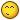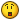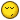## Recommended Posts

Suppose that a Time Machine worked in the same fashion as rewinding a tape, where it made events occur in the opposite order that they happened. Now, suppose that the way it reversed the order of events was by 'reversing' the laws of motion--as an example, when Object A runs into Object B, the force is transferred in the direction Object A came from, instead of transferring in the direction that Object A is moving in. There's actually a name for this in theoretical physics, called Negative Mass.

Therefore (if you follow), this time machine would make the positive mass into negative mass to reverse the order of events. The important thing to recognize here is that if a time machine could work in this way, then it would not be 'traveling to the past' as we normally perceive. Instead, the time machine is getting us to the same instance of events that the past was at. To illustrate, we could 'rewind' events to the same instance as 1950 from 2014 in a matter of 10 years, but the date won't become 1950--instead (whether it is realized or not) it's 2024. For this reason, a paradox cannot occur--the past has already been written. It's a copy of the past (much like a parallel universe) that will be effected by the events after time traveling.

Please let me know what you think. Thanks!

Edited by ThreeEasyWords

##### Share on other sites

"Suppose that a Time Machine worked in the same fashion as rewinding a tape"

You have an idea that is more suited to a story line for a science fiction book or a movie. In that application you can write in as much techno-babel as is needed to carry the technology you create to accomplish the time travel of your idea.

But here your idea must have a mechanism that is in complete agreement with the laws that the universe currently operates by.

And I am quite sure it is Blu-ray.

##### Share on other sites

You have an idea that is more suited to a story line for a science fiction book or a movie. In that application you can write in as much techno-babel as is needed to carry the technology you create to accomplish the time travel of your idea.

But here your idea must have a mechanism that is in complete agreement with the laws that the universe currently operates by.

And I am quite sure it is Blu-ray.

Blu-ray, huh? XD Thanks for respondingI want to propose the idea more as a theory than a story, though, so any advice? Assuming that it were possible to convert positive mass to negative mass, would this conversion reverse the sequence of events? Or would things play out differently than that?

Edited by ThreeEasyWords

##### Share on other sites

Blu-ray, huh? XD Thanks for respondingI want to propose the idea more as a theory then a story, though, so any advice? Assuming that it were possible to convert positive mass to negative mass, would this conversion reverse the sequence of events? Or would things play out differently than that?

See what happens with the laws of physics when you convert positive mass to negative mass.

##### Share on other sites

See what happens with the laws of physics when you convert positive mass to negative mass.

Thanks, I've been thinking about it. I've thought of a number of instances with negative mass, and as far as I can tell, it works.

Taking it a step further, I've been wondering about negative mass and gravity. There's a number of formal analogies between our present understanding of gravity and electromagnetism. Because of this, I'm wondering if mass could work like electrical charge, where opposite 'masses' attract. If that's the case, then couldn't gravity be the attraction of positive mass to negative mass? There would need to be a lot of it if that were the case, so I'm wondering if it's dark matter.

##### Share on other sites

Why would you rewind time by having negative mass?

Taking it a step further, I've been wondering about negative mass and gravity.

The only thing that happens is that negative mass repels positive mass and attracts other negative masses. It does not do anything different other then that.

when Object A runs into Object B, the force is transferred in the direction Object A came from, instead of transferring in the direction that Object A is moving in. There's actually a name for this in theoretical physics, called Negative Mass.

It wouldn't be called negative mass. Negative mass would cause this to happen, but there can be other things, too. For example, if an object ran into a spring, the force would towards the object, without requiring negative mass.

##### Share on other sites

Why would you rewind time by having negative mass?

The only thing that happens is that negative mass repels positive mass and attracts other negative masses. It does not do anything different other then that.

F=ma, so -F = -m * a. If mass becomes negative, then so does force. That's negative force. In simple terms, negative force is force in the opposite direction. That's why events would occur in the opposite direction.

Think about it like this: Ball A is rolled over a table, hitting Ball B. Afterwards, the mass of everything becomes negative. Now, Ball B has negative force, sending it in the direction that it was leaving. The ball collides with Ball A, and Ball A is returned to its starting point. The instance at the start of the scenario has been restored--thus, Ball A and B 'rewinded' into the past.

We don't usually consider 'changing' positive mass to negative, so I can understand the confusion. Such an event isn't commonly pondered about. Correct me if I'm still wrong about it.In reference to the conjecture about gravity, our current understanding of it is incomplete. More pieces are needed. The idea mentioned is meant as a proposal to fill these missing pieces, without going against our current experiments.

The question is whether it does this. There's certainly mathematical work that has to be done to see how it holds up--for now we'll look into proof of concept, though, since this is speculation. Might it work with what we know?

I believe it may--it explains things in more depth than the General Theory of Relativity by itself, without removing its idea. The interactions of negative mass with positive mass would cause the curvature of space-time. Also, the more positive mass, the more negative mass would be attracted. In turn, groups of positive mass would cling to the negative, coming closer to each other in the process. In this way, attraction would be increased between positive masses, indirectly due to their mass. This makes the relationship between positive mass and gravity less precise but present, explaining the problems Dark Matter was meant to fix. A lot of math will need to be looked at for this.

In a more abstract sense, understanding gravity as 'opposites attracting' would fortify its relations to electromagnetism, which is an important problem in Physics. Gravity and magnetism are similar, so its worth considering whether their functions hold similarities, as well. I'd like to here any more ideas, really.As for the last part of your post, you're completely right.I should have said that 'rewinding' is being described through the behavior of negative mass, instead of negative mass itself.

Edited by ThreeEasyWords

##### Share on other sites

F=ma, so -F = -m * a. If mass becomes negative, then so does force. That's negative force. In simple terms, negative force is force in the opposite direction. That's why events would occur in the opposite direction.

Think about it like this: Ball A is rolled over a table, hitting Ball B. Afterwards, the mass of everything becomes negative. Now, Ball B has negative force, sending it in the direction that it was leaving. The ball collides with Ball A, and Ball A is returned to its starting point. The instance at the start of the scenario has been restored--thus, Ball A and B 'rewinded' into the past.

Retracing an event isn't "rewinding" time. The arrow of time is often tied in with entropy. What happens to entropy with negative mass?

##### Share on other sites

Retracing an event isn't "rewinding" time. The arrow of time is often tied in with entropy. What happens to entropy with negative mass?

That's an important point.There would have to be reverse entropy as well.

My question is if entropy is tied with force? If my understanding is correct, its fundamentally a type of force that increases entropy. If that's true, then the negative force of negative mass would seem to cause the opposite. I might be simplifying things a little too much, however. After all, reverse entropy is a problem for a reason.As to the part about retracing an event: if all events are retraced exactly the same, then time may be considered "rewinded". It would appear that way to everyone. Does that make sense?

You are right in saying that retracing a single event wouldn't rewind time correctly--it would have to be the universe as a whole, including entropy. Otherwise we would be having some strange effects.

Edited by ThreeEasyWords

## Create an account

Register a new account